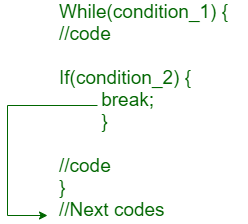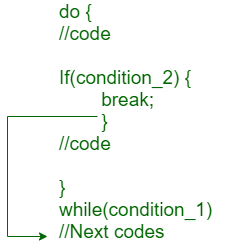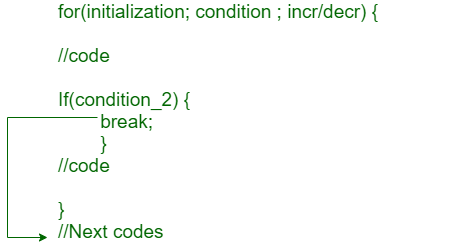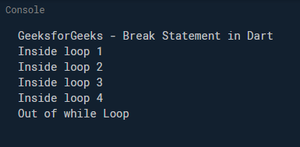Skip to content
Related Articles
Dart – Break Statement
• Last Updated : 15 Jul, 2020

The break statement in Dart inside any loop gives you a way to break or terminate the execution of the loop containing it, and hence transfers the execution to the next statement following the loop. It is always used with the if-else construct.

### Dart Break Statement Flow Diagram

#### While Loop:#### Do While Loop:#### For Loop:```Syntax: break;
```

Example 1:

## Dart

 `// Dart program in Dart to ``// illustrate Break statement ``void` `main()``{``  ``var count = 0;``  ``print(``"GeeksforGeeks - Break Statement in Dart"``);``   ` `  ``while``(count<=10)``  ``{``    ``count++;``    ``if``(count == 5)``    ``{``      ``//break statement``      ``break``; ``    ``}``     ` `    ``print(``"Inside loop \${count}"``);``  ``}``  ``print(``"Out of while Loop"``);``}`

Output:In this above program, the variable count is initialized as 0. Then a while loop is executed as long as the variable count is less than 10.

Inside the while loop, the count variable is incremented by 1 with each iteration (count = count + 1). Next, we have an If statement that checks the variable count is equal to 5, if it returns TRUE causes loop to break or terminate, Within the loop, there is a cout statement that will execute with each iteration of the while loop until the loop breaks. Then, there is a final cout statement outside of the while loop.

Example 2:

## Dart

 `// Dart program in Dart to ``// illustrate Break statement ``void` `main() { ``   ``var i = 1; ``   ``while``(i<=10) { ``      ``if` `(i %  == 0) { ``         ``print(``"The first multiple of 2  between 1 and 10 is : \${i}"``); ``         ``break` `;    ``         ``// exit the loop if the ``         ``// first multiple is found ``      ``} ``      ``i++; ``   ``}``}`

Output:

```The first multiple of 5 between 1 and 10 is: 2
```

My Personal Notes arrow_drop_up